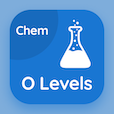Computer Science Online Courses

PHP MCQs

PHP MCQ PDF - Topics

# Mathematical Operator MCQ Quiz Online

Practice Mathematical Operator Multiple Choice Questions (MCQ), Mathematical Operator quiz answers PDF to learn php online course for php classes. PHP Number Handling Multiple Choice Questions and Answers (MCQs), Mathematical Operator quiz questions for software engineering online courses. "Mathematical Operator MCQ" PDF Book: numerical types, mathematical operator, simple mathematical functions, randomness test prep for online computer science and engineering.

"Which operators have higher precedence?" MCQ PDF: mathematical operator with choices arithmetic operators, comparison operators, logical operators, and boolean operators for software engineering online courses. Learn mathematical operator quiz questions for merit scholarship test and certificate programs for top computer science schools.

## MCQs on Mathematical Operator Quiz

MCQ: Which operators have higher precedence?

Arithmetic operators
Comparison operators
Logical operators
Boolean operators

MCQ: The decrement operator is denoted by

++
−−
%%
⁄⁄

MCQ: Comparison operators have higher precedence than

Arithmetic operators
Assignment operators
Boolean operators
All of them

MCQ: The === operator is true if its two arguments are exactly

Equal
Opposite
Has the same type
Both A and C

MCQ: The increment operator is denoted by

++
−−
%%
⁄⁄

### More Topics from PHP Course